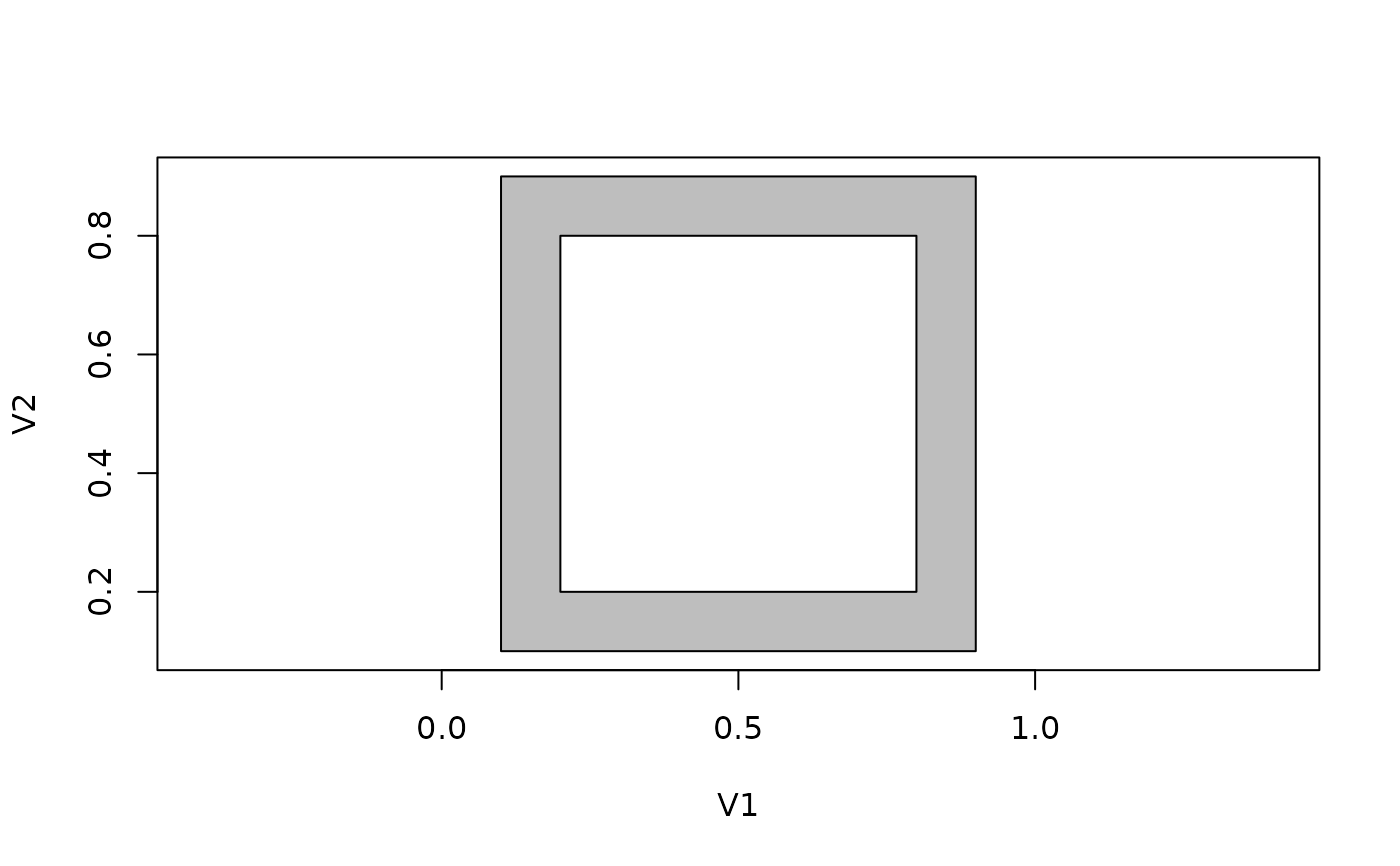Plot the triangles produced by earcut, or plot the polygon paths using the same interface as earcut uses. This allows for easy comparison and checking of what the results should be.

plot_ears(xy, idx, add = FALSE, ...)

plot_holes(xy, holes = 0, add = FALSE, ...)

## Arguments

xy xy-coordinates, either a list, matrix, or data frame index of triangles add to current plot, or create a new arguments to polypath index of starting position of holes (see earcut)

## Details

For both functions the first input is a matrix of x,y coordinates.

For plot_ears the second input is the index output of earcut. The index is treated in sets of 3 values, with individual calls to polypath to draw a polygon for each triangle.

For plot_holes the second input is the holes argument that would be used for earcut. This is used to split the coordinates at these positions, inserting NA values as per the mechanism used by graphics::polypath to break coordinates into separate polygon rings. (There's no winding rule here plot_rules is hard-coded to always use the evenodd rule, so that winding order may be ignored).

## after ?polypath
plot_holes(x, holes = 5, col = "grey")